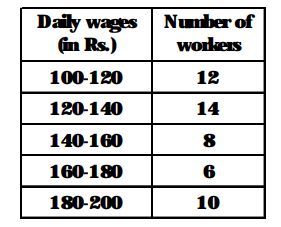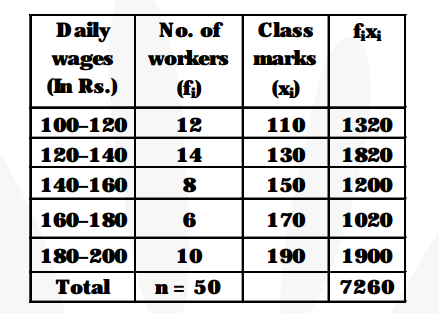# Consider the following distribution of daily wages of 50 workers of a factory.Question:

Consider the following distribution of daily wages of 50 workers of a factory.Find the mean daily wages of the workers of the factory by using an appropriate method.

Solution:We have $\Sigma f_{i}=50$ and $\sum f_{i} x_{i}=7260$

Mean $=\frac{\sum \mathbf{f}_{\mathbf{x}} \mathbf{i}}{\sum \mathbf{f}}=\frac{\mathbf{7 2 6 0}}{\mathbf{5 0}}=145.2$